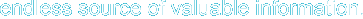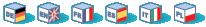# Integers

An integer is a number of the set Z = {..., -2, -1, 0, 1, 2, ...}.

## Syntax

Integers can be specified in decimal (10-based), hexadecimal (16-based) or octal (8-based) notation, optionally preceded by a sign (- or +).

If you use the octal notation, you must precede the number with a 0 (zero), to use hexadecimal notation precede the number with 0x.

Esempio 11-1. Integer literals

 ``` ```
Formally the possible structure for integer literals is:

 ```decimal : [1-9][0-9]* | 0 hexadecimal : 0[xX][0-9a-fA-F]+ octal : 0[0-7]+ integer : [+-]?decimal | [+-]?hexadecimal | [+-]?octal```

The size of an integer is platform-dependent, although a maximum value of about two billion is the usual value (that's 32 bits signed). PHP does not support unsigned integers.

Avvertimento

If an invalid digit is passed to octal integer (i.e. 8 or 9), the rest of the number is ignored.

Esempio 11-2. Octal weirdness

 ``` ```

## Integer overflow

If you specify a number beyond the bounds of the integer type, it will be interpreted as a float instead. Also, if you perform an operation that results in a number beyond the bounds of the integer type, a float will be returned instead.

 ``` ```

 Avvertimento Unfortunately, there was a bug in PHP so that this does not always work correctly when there are negative numbers involved. For example: when you do -50000 * \$million, the result will be -429496728. However, when both operands are positive there is no problem. This is solved in PHP 4.1.0.

There is no integer division operator in PHP. 1/2 yields the float 0.5. You can cast the value to an integer to always round it downwards, or you can use the round() function.

 ``` ```

## Converting to integer

To explicitly convert a value to integer, use either the (int) or the (integer) cast. However, in most cases you do not need to use the cast, since a value will be automatically converted if an operator, function or control structure requires an integer argument. You can also convert a value to integer with the function intval().

### From booleans

FALSE will yield 0 (zero), and TRUE will yield 1 (one).

### From floating point numbers

When converting from float to integer, the number will be rounded towards zero.

If the float is beyond the boundaries of integer (usually +/- 2.15e+9 = 2^31), the result is undefined, since the float hasn't got enough precision to give an exact integer result. No warning, not even a notice will be issued in this case!

Avvertimento

Never cast an unknown fraction to integer, as this can sometimes lead to unexpected results.

 ``` ```